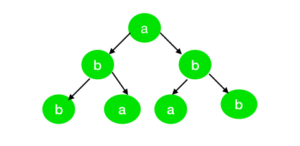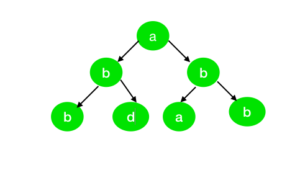Related Articles

# Check if Inorder traversal of a Binary Tree is palindrome or not

• Last Updated : 18 Jun, 2021

Given a binary tree and the task if to check if its Inorder Sequence is a palindrome or not.
Examples:

Input:Output: True
Explanation:
The Inorder sequence of the tree is “bbaaabb” which is a palindromic string.
Input:Output: False
Explanation:
The Inorder sequence of the tree is “bbdaabb” which is not a palindromic string.

Approach:
To solve the problem, refer to the following steps:

Below is the implementation of above approach:

## C++

 `// C++ Program to check if``// the Inorder sequence of a``// Binary Tree is a palindrome` `#include ``using` `namespace` `std;` `// Define node of tree``struct` `node {``    ``char` `val;``    ``node* left;``    ``node* right;``};` `// Function to create the node``node* newnode(``char` `i)``{``    ``node* temp = NULL;``    ``temp = ``new` `node();``    ``temp->val = i;``    ``temp->left = NULL;``    ``temp->right = NULL;``    ``return` `temp;``}` `// Function to convert the tree``// to the double linked list``node* conv_tree(node* root,``                ``node* shoot)``{``    ``if` `(root->left != NULL)``        ``shoot = conv_tree(root->left,``                          ``shoot);` `    ``root->left = shoot;` `    ``if` `(shoot != NULL)``        ``shoot->right = root;` `    ``shoot = root;` `    ``if` `(root->right != NULL)``        ``shoot = conv_tree(root->right,``                          ``shoot);` `    ``return` `shoot;``}` `// Function for checking linked``// list is palindrom or not``int` `checkPalin(node* root)``{``    ``node* voot = root;` `    ``// Count the nodes``    ``// form the back``    ``int` `j = 0;` `    ``// Set the pointer at the``    ``// very first node of the``    ``// linklist and count the``    ``// length of the linklist``    ``while` `(voot->left != NULL) {``        ``j = j + 1;``        ``voot = voot->left;``    ``}``    ``int` `i = 0;` `    ``while` `(i < j) {``        ``// Check if the value matches``        ``if` `(voot->val != root->val)``            ``return` `0;` `        ``else` `{``            ``i = i + 1;``            ``j = j - 1;``            ``voot = voot->right;``            ``root = root->left;``        ``}``    ``}` `    ``return` `1;``}` `// Driver Program``int` `main()``{``    ``node* root = newnode(``'b'``);``    ``root->left = newnode(``'b'``);``    ``root->right = newnode(``'a'``);``    ``root->left->right = newnode(``'b'``);``    ``root->left->left = newnode(``'a'``);` `    ``node* shoot = conv_tree(root, NULL);` `    ``if` `(checkPalin(shoot))``        ``cout << ``"True"` `<< endl;``    ``else``        ``cout << ``"False"` `<< endl;``}`

## Java

 `// Java Program to check if``// the Inorder sequence of a``// Binary Tree is a palindrome``import` `java.util.*;``class` `GFG{` `// Define node of tree``static` `class` `node``{``    ``char` `val;``    ``node left;``    ``node right;``};` `// Function to create the node``static` `node newnode(``char` `i)``{``    ``node temp = ``null``;``    ``temp = ``new` `node();``    ``temp.val = i;``    ``temp.left = ``null``;``    ``temp.right = ``null``;``    ``return` `temp;``}` `// Function to convert the tree``// to the double linked list``static` `node conv_tree(node root,``                      ``node shoot)``{``    ``if` `(root.left != ``null``)``        ``shoot = conv_tree(root.left,``                          ``shoot);` `    ``root.left = shoot;` `    ``if` `(shoot != ``null``)``        ``shoot.right = root;` `    ``shoot = root;` `    ``if` `(root.right != ``null``)``        ``shoot = conv_tree(root.right,``                          ``shoot);` `    ``return` `shoot;``}` `// Function for checking linked``// list is palindrom or not``static` `int` `checkPalin(node root)``{``    ``node voot = root;` `    ``// Count the nodes``    ``// form the back``    ``int` `j = ``0``;` `    ``// Set the pointer at the``    ``// very first node of the``    ``// linklist and count the``    ``// length of the linklist``    ``while` `(voot.left != ``null``)``    ``{``        ``j = j + ``1``;``        ``voot = voot.left;``    ``}``    ``int` `i = ``0``;` `    ``while` `(i < j)``    ``{``        ``// Check if the value matches``        ``if` `(voot.val != root.val)``            ``return` `0``;` `        ``else``        ``{``            ``i = i + ``1``;``            ``j = j - ``1``;``            ``voot = voot.right;``            ``root = root.left;``        ``}``    ``}``    ``return` `1``;``}` `// Driver Code``public` `static` `void` `main(String[] args)``{``    ``node root = newnode(``'b'``);``    ``root.left = newnode(``'b'``);``    ``root.right = newnode(``'a'``);``    ``root.left.right = newnode(``'b'``);``    ``root.left.left = newnode(``'a'``);` `    ``node shoot = conv_tree(root, ``null``);` `    ``if` `(checkPalin(shoot) == ``1``)``        ``System.out.print(``"True"` `+ ``"\n"``);``    ``else``        ``System.out.print(``"False"` `+ ``"\n"``);``}``}` `// This code is contributed by 29AjayKumar`

## Python3

 `# Python3 program to check if``# the Inorder sequence of a``# Binary Tree is a palindrome` `# Define node of tree``class` `Node:``    ``def` `__init__(``self``,val):``        ` `        ``self``.val ``=` `val``        ``self``.left ``=` `None``        ``self``.right ``=` `None` `# Function to create the node``def` `newnode(i):` `    ``#temp = None;``    ``temp ``=` `Node(i);``    ``temp.val ``=` `i;``    ``temp.left ``=` `None``;``    ``temp.right ``=` `None``;``    ``return` `temp;` `# Function to convert the tree``# to the double linked list``def` `conv_tree(root, shoot):` `    ``if` `(root.left !``=` `None``):``        ``shoot ``=` `conv_tree(root.left, shoot);` `    ``root.left ``=` `shoot;` `    ``if` `(shoot !``=` `None``):``        ``shoot.right ``=` `root;` `    ``shoot ``=` `root;` `    ``if` `(root.right !``=` `None``):``        ``shoot ``=` `conv_tree(root.right, shoot);` `    ``return` `shoot;` `# Function for checking linked``# list is palindrom or not``def` `checkPalin(root):` `    ``voot ``=` `root;` `    ``# Count the nodes``    ``# form the back``    ``j ``=` `0``;` `    ``# Set the pointer at the``    ``# very first node of the``    ``# linklist and count the``    ``# length of the linklist``    ``while` `(voot.left !``=` `None``):``        ``j ``=` `j ``+` `1``;``        ``voot ``=` `voot.left;``    ` `    ``i ``=` `0``;` `    ``while` `(i < j):``        ` `        ``# Check if the value matches``        ``if` `(voot.val !``=` `root.val):``            ``return` `0``;``        ``else``:``            ``i ``=` `i ``+` `1``;``            ``j ``=` `j ``-` `1``;``            ``voot ``=` `voot.right;``            ``root ``=` `root.left;``            ` `    ``return` `1``;` `# Driver code``if` `__name__``=``=``'__main__'``:` `    ``root ``=` `newnode(``'b'``);``    ``root.left ``=` `newnode(``'b'``);``    ``root.right ``=` `newnode(``'a'``);``    ``root.left.right ``=` `newnode(``'b'``);``    ``root.left.left ``=` `newnode(``'a'``);` `    ``shoot ``=` `conv_tree(root, ``None``);` `    ``if` `(checkPalin(shoot)):``        ``print``(``"True"``);``    ``else``:``        ``print``(``"False"``);` `# This code is contributed by AbhiThakur`

## C#

 `// C# program to check if the inorder ``// sequence of a Binary Tree is a``// palindrome``using` `System;` `class` `GFG{` `// Define node of tree``class` `node``{``    ``public` `char` `val;``    ``public` `node left;``    ``public` `node right;``};` `// Function to create the node``static` `node newnode(``char` `i)``{``    ``node temp = ``null``;``    ``temp = ``new` `node();``    ``temp.val = i;``    ``temp.left = ``null``;``    ``temp.right = ``null``;` `    ``return` `temp;``}` `// Function to convert the tree``// to the double linked list``static` `node conv_tree(node root,``                      ``node shoot)``{``    ``if` `(root.left != ``null``)``        ``shoot = conv_tree(root.left,``                          ``shoot);` `    ``root.left = shoot;` `    ``if` `(shoot != ``null``)``        ``shoot.right = root;` `    ``shoot = root;` `    ``if` `(root.right != ``null``)``        ``shoot = conv_tree(root.right,``                          ``shoot);` `    ``return` `shoot;``}` `// Function for checking linked``// list is palindrom or not``static` `int` `checkPalin(node root)``{``    ``node voot = root;` `    ``// Count the nodes``    ``// form the back``    ``int` `j = 0;` `    ``// Set the pointer at the``    ``// very first node of the``    ``// linklist and count the``    ``// length of the linklist``    ``while` `(voot.left != ``null``)``    ``{``        ``j = j + 1;``        ``voot = voot.left;``    ``}``    ``int` `i = 0;` `    ``while` `(i < j)``    ``{``        ` `        ``// Check if the value matches``        ``if` `(voot.val != root.val)``            ``return` `0;``        ``else``        ``{``            ``i = i + 1;``            ``j = j - 1;``            ``voot = voot.right;``            ``root = root.left;``        ``}``    ``}``    ``return` `1;``}` `// Driver Code``public` `static` `void` `Main(String[] args)``{``    ``node root = newnode(``'b'``);``    ``root.left = newnode(``'b'``);``    ``root.right = newnode(``'a'``);``    ``root.left.right = newnode(``'b'``);``    ``root.left.left = newnode(``'a'``);` `    ``node shoot = conv_tree(root, ``null``);` `    ``if` `(checkPalin(shoot) == 1)``        ``Console.Write(``"True"` `+ ``"\n"``);``    ``else``        ``Console.Write(``"False"` `+ ``"\n"``);``}``}` `// This code is contributed by 29AjayKumar`

## Javascript

 ``
Output:
`True`

Time Complexity: O(N)
Auxiliary Space: O(1)

Attention reader! Don’t stop learning now. Get hold of all the important DSA concepts with the DSA Self Paced Course at a student-friendly price and become industry ready.  To complete your preparation from learning a language to DS Algo and many more,  please refer Complete Interview Preparation Course.

In case you wish to attend live classes with experts, please refer DSA Live Classes for Working Professionals and Competitive Programming Live for Students.

My Personal Notes arrow_drop_up